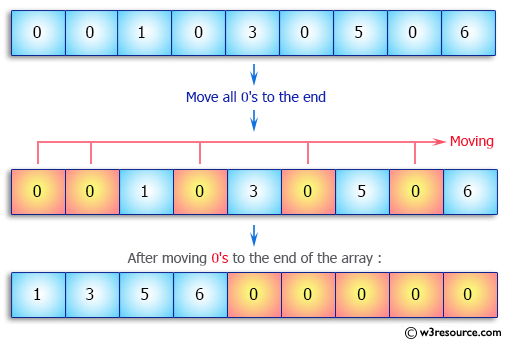﻿ Java exercises: Move all 0's to the end of an array - w3resource# Java Array Exercises: Move all 0's to the end of an array

## Java Array: Exercise-26 with Solution

Write a Java program to move all 0's to the end of an array. Maintain the relative order of the other (non-zero) array elements.

Pictorial Presentation:Sample Solution:

Java Code:

``````import java.util.*;
public class Exercise26 {
public static void main(String[] args) throws Exception {
int[] array_nums = {0,0,1,0,3,0,5,0,6};
int i = 0;
System.out.print("\nOriginal array: \n");
for (int n : array_nums)
System.out.print(n+"  ");

for(int j = 0, l = array_nums.length; j < l;) {
if(array_nums[j] == 0)
j++;
else {
int temp = array_nums[i];
array_nums[i] = array_nums[j];
array_nums[j] = temp;
i ++;
j ++;
}
}
while (i < array_nums.length)
array_nums[i++] = 0;
System.out.print("\nAfter moving 0's to the end of the array: \n");
for (int n : array_nums)
System.out.print(n+"  ");
System.out.print("\n");
}
}
```
```

Sample Output:

```
Original array:
0  0  1  0  3  0  5  0  6
After moving 0's to the end of the array:
1  3  5  6  0  0  0  0  0
```

Flowchart:Visualize Java code execution (Python Tutor):

Java Code Editor:

Improve this sample solution and post your code through Disqus

What is the difficulty level of this exercise?

Test your Programming skills with w3resource's quiz.

﻿

## Java: Tips of the Day

countOccurrences

Counts the occurrences of a value in an array.

Use Arrays.stream().filter().count() to count total number of values that equals the specified value.

```public static long countOccurrences(int[] numbers, int value) {
return Arrays.stream(numbers)
.filter(number -> number == value)
.count();
}
```

Ref: https://bit.ly/3kCAgLb